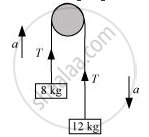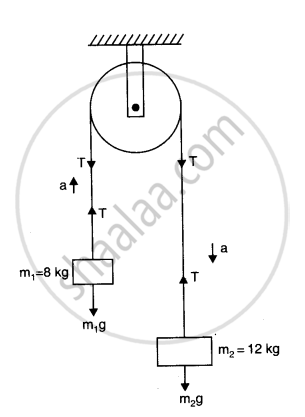CBSE (Science) Class 11CBSE
Share

# Two Masses 8 Kg and 12 Kg Are Connected at the Two Ends of a Light Inextensible String that Goes Over a Frictionless Pulley. Find the Acceleration of the Masses, and the Tension in the String When the Masses Are Released. - CBSE (Science) Class 11 - Physics

ConceptNewton’s Second Law of Motion

#### Question

Two masses 8 kg and 12 kg are connected at the two ends of a light inextensible string that goes over a frictionless pulley. Find the acceleration of the masses, and the tension in the string when the masses are released.

#### Solution 1

The given system of two masses and a pulley can be represented as shown in the following figure:Smaller mass, m1 = 8 kg

Larger mass, m2 = 12 kg

Tension in the string = T

Mass m2, owing to its weight, moves downward with acceleration a,and mass m1moves upward.

Applying Newton’s second law of motion to the system of each mass:

For mass m1:

The equation of motion can be written as:

T – m1g = ma … (i)

For mass m2:

The equation of motion can be written as:

m2g – T = m2a … (ii)

Adding equations (i) and (ii), we get:

(m_2-m_1)g = (m_1+ m_2)a

:.a = ((m_2-m_1)/(m_1+m_2))g ...(iii)

=((12-8)/(12+8)) xx 10= 4/20 xx 10 = 2 "m/s"^2

Therefore, the acceleration of the masses is 2 m/s2.

Substituting the value of a in equation (ii), we get:

m_2g - T = m_2 ( (m_2-m_1)/(m_1+m_2))g

=((2m_1m_2)/(m_1+m_2))g

=(2xx12xx8)/(12+8)xx10

=(2xx12xx8)/20 xx 10  = 96 N

Therefore, the tension in the string is 96 N.

#### Solution 2

For block m_2 -> m_2g - T = m_2a  ...(i)

and for block m_1 -> T - m_1g = m_1a  ...(ii)

Adding i and ii we obtain

(m_2 - m_1)g = (m_2+m_1)a

or a = ((m_2-m_1)/(m_2+m_1))g

= (12 - 8)/(12 + 8) xx 10

= (4xx10)/20 = 2 ms^(-2)

Substituting value of a in equation ii we obtain

T = m_1(g+a)

= 8 xx (10 + 2)

= 8 x12 = 96 NIs there an error in this question or solution?

#### APPEARS IN

Solution Two Masses 8 Kg and 12 Kg Are Connected at the Two Ends of a Light Inextensible String that Goes Over a Frictionless Pulley. Find the Acceleration of the Masses, and the Tension in the String When the Masses Are Released. Concept: Newton’s Second Law of Motion.
S